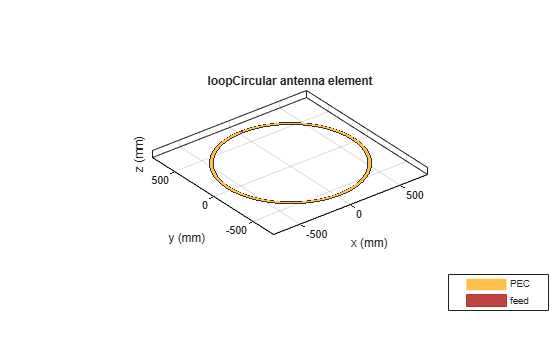# loopCircular

Create circular loop antenna

## Description

The `loopCircular` object is a planar circular loop antenna on the xy- plane.

The thickness of the loop is related to the diameter of an equivalent cylinder loop by the equation

`$t=2d=4r$`

, where:

• d is the diameter of equivalent cylindrical loop

• r is the radius of equivalent cylindrical loop

For a given cylinder radius, use the `cylinder2strip` utility function to calculate the equivalent width. The default circular loop antenna is fed at the positive x-axis. The point of the x-axis is at the midpoint of the inner and outer radii.## Creation

### Syntax

``lc = loopCircular``
``lc = loopCircular(Name,Value)``

### Description

``` `lc = loopCircular` creates a one wavelength circular loop antenna in the X-Y plane. By default, the circumference is chosen for the operating frequency 75 MHz.```

example

``` `lc = loopCircular(Name,Value)` creates a one wavelength circular loop antenna, with additional properties specified by one, or more name-value pair arguments. `Name` is the property name and `Value` is the corresponding value. You can specify several name-value pair arguments in any order as `Name1`, `Value1`, `...`, `NameN`, `ValueN`. Properties not specified retain their default values.```

## Properties

expand all

Outer radius of loop, specified as a scalar in meters.

Example: `'Radius',3`

Data Types: `double`

Thickness of loop, specified as a scalar in meters.

Example: `'Thickness',2`

Data Types: `double`

Type of the metal used as a conductor, specified as a metal material object. You can choose any metal from the `MetalCatalog` or specify a metal of your choice. For more information, see `metal`. For more information on metal conductor meshing, see Meshing.

Example: ```m = metal('Copper'); 'Conductor',m```

Example: ```m = metal('Copper'); ant.Conductor = m```

Lumped elements added to the antenna feed, specified as a lumped element object. For more information, see `lumpedElement`.

Example: `'Load',lumpedelement`. `lumpedelement` is the object for the load created using `lumpedElement`.

Example: ```lc.Load = lumpedElement('Impedance',75)```

Tilt angle of the antenna in degrees, specified as a scalar or vector. For more information, see Rotate Antennas and Arrays.

Example: `90`

Example: `Tilt=[90 90]`,`TiltAxis=[0 1 0;0 1 1]` tilts the antenna at 90 degrees about the two axes defined by the vectors.

Data Types: `double`

Tilt axis of the antenna, specified as one of these values:

• Three-element vector of Cartesian coordinates in meters. In this case, each coordinate in the vector starts at the origin and lies along the specified points on the x-, y-, and z-axes.

• Two points in space, specified as a 2-by-3 matrix corresponding to two three-element vectors of Cartesian coordinates. In this case, the antenna rotates around the line joining the two points.

• `"x"`, `"y"`, or `"z"` to describe a rotation about the x-, y-, or z-axis, respectively.

Example: `[0 1 0]`

Example: `[0 0 0;0 1 0]`

Example: `"Z"`

Data Types: `double` | `string`

## Object Functions

 `show` Display antenna, array structures, shapes, or platform `info` Display information about antenna or array `axialRatio` Axial ratio of antenna `beamwidth` Beamwidth of antenna `charge` Charge distribution on antenna or array surface `current` Current distribution on antenna or array surface `design` Design prototype antenna or arrays for resonance around specified frequency or create AI-based antenna from antenna catalog objects `efficiency` Radiation efficiency of antenna `EHfields` Electric and magnetic fields of antennas or embedded electric and magnetic fields of antenna element in arrays `impedance` Input impedance of antenna or scan impedance of array `mesh` Mesh properties of metal, dielectric antenna, or array structure `meshconfig` Change meshing mode of antenna, array, custom antenna, custom array, or custom geometry `optimize` Optimize antenna or array using SADEA optimizer `pattern` Plot radiation pattern and phase of antenna or array or embedded pattern of antenna element in array `patternAzimuth` Azimuth plane radiation pattern of antenna or array `patternElevation` Elevation plane radiation pattern of antenna or array `rcs` Calculate and plot radar cross section (RCS) of platform, antenna, or array `returnLoss` Return loss of antenna or scan return loss of array `sparameters` Calculate S-parameters for antennas and antenna arrays `vswr` Voltage standing wave ratio (VSWR) of antenna or array element

## Examples

collapse all

Create and view a circular loop with 0.65 m radius and 0.01 m thickness.

```c = loopCircular('Radius',0.64,'Thickness',0.03); show(c)```Calculate the impedance of a circular loop antenna over a frequency range of 70MHz-90MHz.

```c = loopCircular('Radius',0.64,'Thickness',0.03); impedance(c,linspace(70e6,90e6,31))```Balanis, C.A. Antenna Theory. Analysis and Design, 3rd Ed. New York: Wiley, 2005.